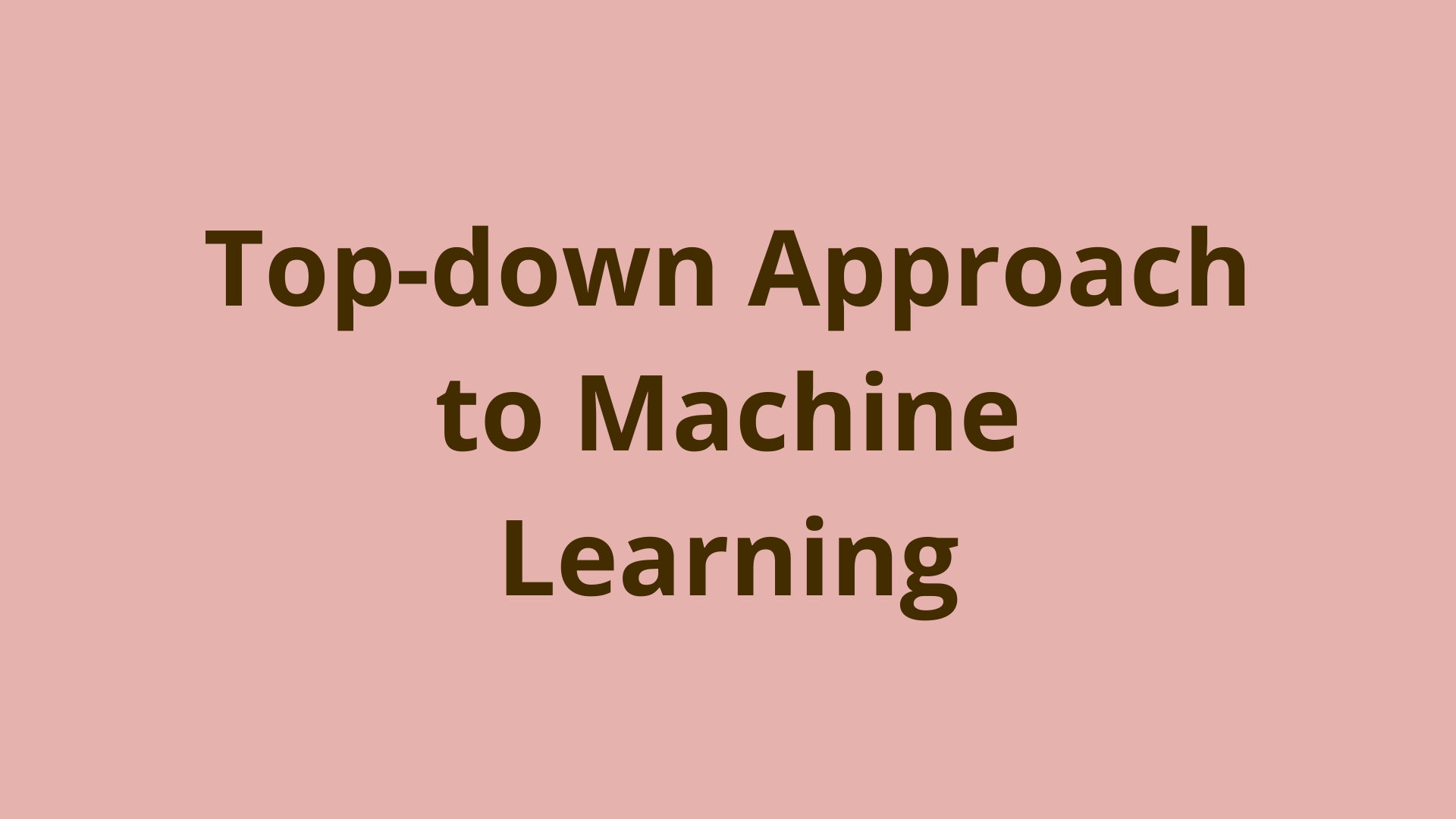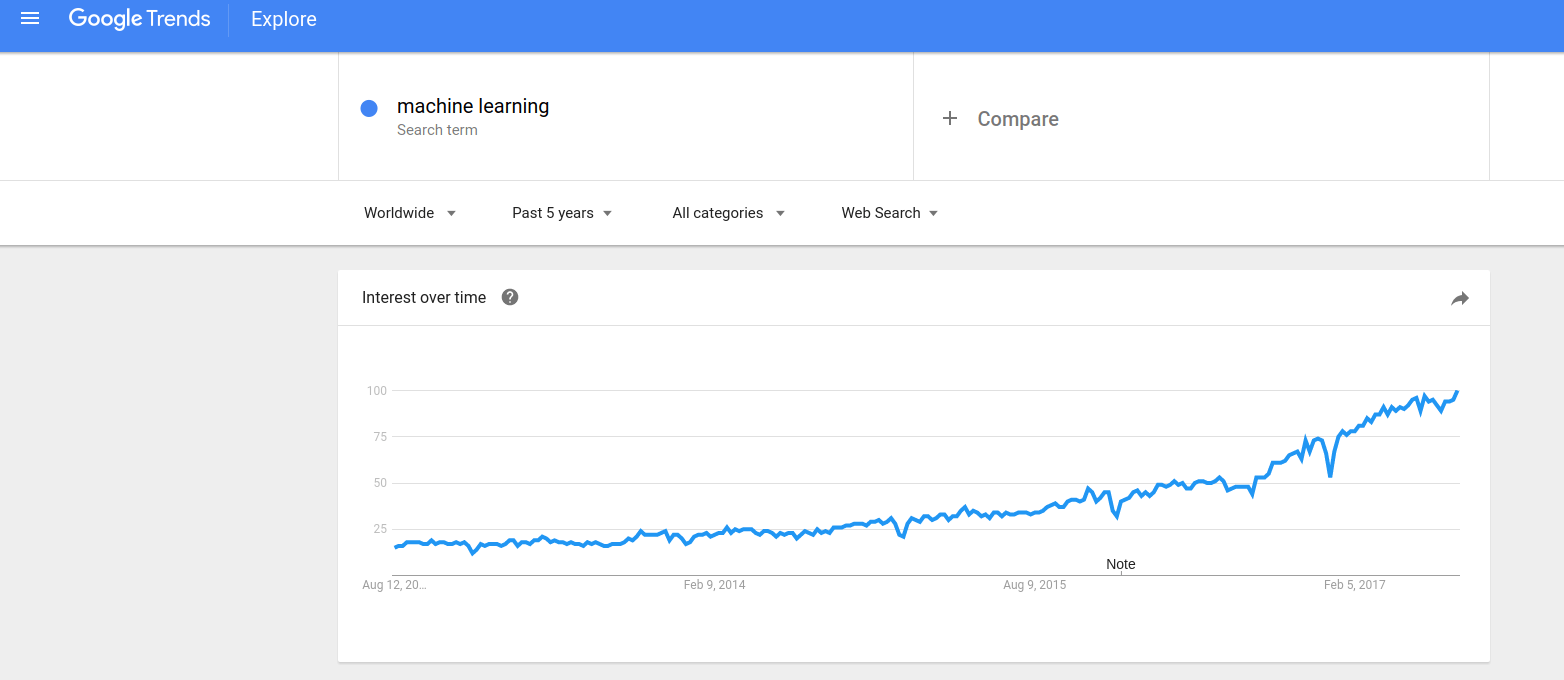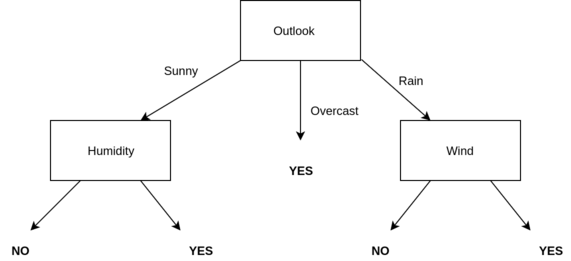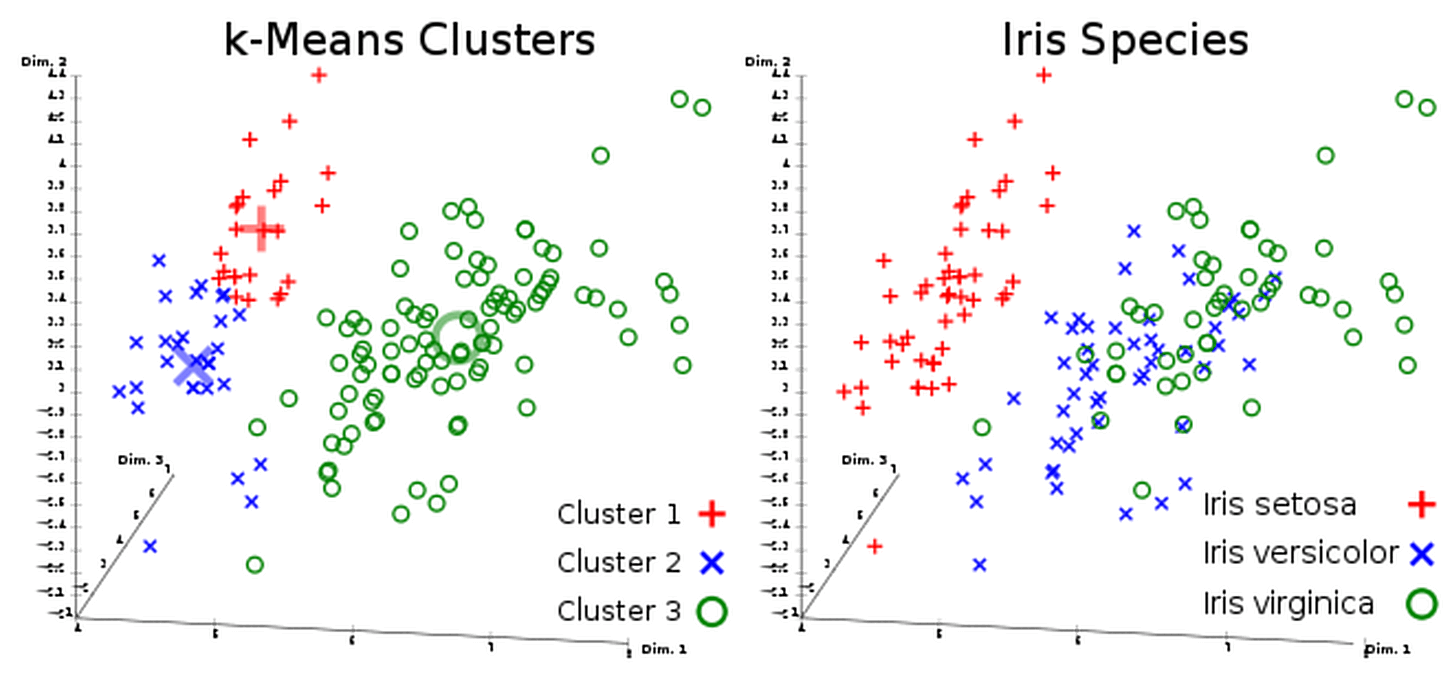## Introduction

Machine Learning (ML) has some hefty gravitational force in the Software development world at the moment. But what exactly is it? In this post, I'll take a top-down approach attempting to make it crystal clear, what it is, and what it can be used for in the real world. Machine Learning is a branch of Artificial Intelligence. Fundamentally it is Software that works like our brain, learning from information (data), then applying it to make smart decisions. Machine Learning algorithms can improve software (a robot) and it's the ability to solve problems through gaining experience. Somewhat like human memory. Whether you know it, or not, you're probably already using applications that leverage Machine Learning algorithms.

Applications might be monitoring your behavior to give you more * personalized* content. A simple example... Google uses Machine Learning in their "Search" product to predict what you might want to search for next. Remember too, it is likely when using the product that the responded suggestions are sometimes entirely inaccurate or not helpful. This is the nature of using a probabilistic method of approach. Some times you hit, and some times you miss.

More and more people are becoming interested in Machine Learning. Companies are adopting it to gauge a better understanding of their clients, which results in providing better customer service. It is being used in streams of gambling, and stock market applications to predict rises and falls of stock prices. Particularly as a Software developer, the demand has become more prominent for skills in the AI and Machine Learning realm. It doesn't look like this trend is slowing down anytime soon. Here is a snapshot of the World's growing interest in ML over the last 5 years...## Foundational learning

The term Agent is commonly referred to in AI, representing a type of computer program. What makes it different from other computer programs? It is a program that gathers information on a particular environment, then takes action(s) autonomously using gathered information. This could be a web crawler, a stock-trading platform, or any other program that can make informed decisions.

How do we define an Agent?

• State-space: The set of all possible states that the agent can be in. For example, the light switch can only ever be "on" or "off".

• Action space: The set of all possible actions that the agent can perform. For example, the light switch can only ever be "flicked up" or "flicked down".

• Percept space: The set of all possible things the agent can perceive in the world. For example, "Fog-of-War in a gaming context, where you can only see what is visible on the map.".

• World dynamics: The change of one state to another, given a particular action. For example, perform the light switch action "flicked up" with the state "off" will result in a change of state to "on".

• Percept function: A change in state results in a new perception of the world. For example, in a gaming context, moving into the enemy base will show you enemy resources.".

• Utility function: The Utility function is used to assign a value to a state. This is can be used to ensure your agent performs an action to land in the best possible state.

This design can be used as a 'structure' to work from and build an AI agent. But you might ask, how does it even relate to Machine Learning? Machine Learning algorithms can enhance the agent to learn better, and perform smarter actions. This is achieved by providing the algorithm with data to learn from and make some smart estimates/predictions. You might be thinking... but wait, can't we just feed it all of the data on the internet to teach it everything? It doesn't exactly work like that. In order for an ML algorithm to learn properly, we need to provide it with the right combination of data, mixed with the right amount of data. Too much, and we might run into an overfitting problem. Too little, and we might have a shitty model that doesn't provide decent predictions.

I also invite you to take a look into the Tree data structure if you aren't familiar with it already. These are used in many modern-day applications.

Ok let's dive headfirst into the 3 major types of algorithms in the field of Machine Learning:

• Supervised learning
• Unsupervised learning
• Reinforcement learning

## Supervised learning

Supervised learning is the name of ML algorithms that learn from examples. This means we must provide the algorithm with training data prior to running the algorithm. An example of this was hilariously shown in the T.V show "Silicon Valley", where a mobile app 'Not hot dog' made media headlines. In the television show, Jian Yang had to provide training data for his ML algorithm to learn from hot dogs. This was to classify if an image of a hot dog was in fact a hotdog, or it was... not a hotdog. How did he do this? In the show, he had to manually scrape the internet for many images of hotdogs (a.k.a the training data).This technique is called Boolean Classification, as a result, itself is a binary value. The algorithm can make a prediction that in the picture there is a hotdog. This was done by analyzing a large number of hotdog pictures and the algorithm has learned to identify what looks like a hotdog. Statistical Classification is used in Supervised learning where the training data is a set of correctly identified observations. For example, in the hotdog scenario, there must only be pictures of hot dogs.

One of the simplest Supervised learning algorithms to implement is Decision Tree. A Decision Tree is where the leaf nodes of the tree are the results, non-leaf nodes are the attributes, and the edges of the tree are the values. The Decision Tree analyses the attributes and returns a result that has been filtered from the tree.Note: As you can see the result in the Decision Tree above can be either "Yes" or "No".

Another technique that is classified as 'Supervised learning' is regression. The simplest form of regression is linear regression, where intuitively this is just drawing a straight line through some data to infer a trend. This could be used in a gambling scenario, analyzing a history of chosen numbers. For example, performing regression on this history might show numbers '5' and '7' are chosen more than numbers '3' and '2'. As we can see below, this is an example of performing regression analysis on some data points...## Unsupervised learning

Unsupervised learning is where the results of the training data are not known. Simply put, we can give the ML algorithm some training data and it can respond back with what the algorithm has found. Sounds exciting! We might receive completely new insights into the data that we would never expect to observe. How is it done? Unsupervised learning commonly uses clustering techniques that aim to find patterns in the data.

One common clustering technique is called "k-means clustering", which aims to solve clustering problems. One common clustering problem is Spam filtering. Spam emails can sometimes be tricky to identify and might get through to your email inbox (instead of the junk folder). The K-means clustering approach aims to partition N observations into K clusters. Essentially, it just moves the spam in the spam cluster and the real emails into the inbox cluster. N is dependent upon the number of emails, and K is dependent upon the number of clusters. Still interested? Here is a study that has shown K-means is a better approach to take than using Support Vector Machines (SVM) in a Spam filtering context.## Hybrid supervised/unsupervised learning

Some ML algorithms can be used for both Supervised and Unsupervised learning. After all, the only dramatic difference between the two is just knowing the end result. The commonly used methods that can be relevant for both cases are Bayesian Networks, Neural Networks, and ...Decision Trees! Yes, that's right, a Supervised learning algorithm can also be used for Unsupervised learning. This is straight-up magic. What we're actually trying to do is run a Supervised algorithm and find an Unsupervised result (a completely new/unexpected result). To do this, we must provide the algorithm with a second group of observations, this way it can recognize the difference between the two observation groups. As a result, the Decision tree can find new clusters by having additional observation groups.

Bayesian Networks utilize graphs, probability theory, and statistics to model real-world situations and infer data insights. These are very compact and can be used for modeling a solution quite quickly. How do we create one? Well, we need...

1. An acyclic graph; and
2. Conditional Probability Tables (CPT)

Shown below is an example graph, and the CPTs given according to the node placements on the graph.Here we can make some simple inferences from the CPTs, such as when it is raining and the sprinkler is turned on, then there is a 99% chance that the grass is wet. Sure, it's a silly example but the point is that we can apply this to more valuable use cases that yield greater results. These can get more complicated by adding extra parent nodes into the equation, also having to estimate probability values in some cases.

A Neural Network is a Machine Learning algorithm capable of simulating the human brain. A Neural network is made up of interconnected artificial neurons. A neuron is basically just a function applied to a linear combination of inputs. Each input is multiplied by a weight, which is essentially a measurement to see how strong the input is for determining the output. By using some math we can represent this as...

$$Y = w_1X_1 + w_2X_2 + w_3X_3 ... + w_nX_n$$

$$A = \frac{1}{1 + e^-Y}$$

Y is the linear combination of inputs, and A is the activation function. Hmm.. can we input just any data into the neuron? Not really. Neural Networks only work with numerical data, which means you cannot initialize a variable like eg. $$X_1 = "Apple"$$. However, it is possible to get around this if we were trying to make predictions on natural language. This gets complicated fast, but the idea being is we encode the string so it can be fed into the Neural network. Here is an example of the "Bag-of-words" model used in Natural Language Processing to encode an array of words to numbers.Above is a Neural network representation. Don't freak out about what everything means. Let us deduce it. The left-hand corner is the input range $$X_1...X_n$$ and the "transfer function" is simply the function to combine all the inputs. It hands the result, in our case Y, to the activation function which computes A. Another aspect to take into account when building a Neural network is the technique used for learning the weights. Calibrating the weights of the Neural network is the "training process". This is done by alternating techniques, "Forward propagation" and "Backpropagation". Forward propagation is how we approached the above equation, applying the weights to the input data before computing the activation function. We received the output and could compare it to real value to get a margin of error (checking if it is what we wanted). Backpropagation is the process of going back through the network to reduce the margin of error.Here we can see a more complicated Neural network with many hidden layers. What are these extra layers even for? Well... our last example only had one layer, which was really to compute a specific function (task-specific). Adding extra layers to the Neural network allows us to learn much more than a specific result, but allows us to classify things from raw data. This process is called Feature learning. This can be used to analyze unstructured data such as images, video, and sensor data. You probably have used applications that have implemented this technique before. Feature learning can be used to identify people, places, things, you name it. There have been great technological advancements recently for Deep learning and Feature learning, especially after the rise of Web 2.0.

## Reinforcement Learning

Reinforcement learning is the learn by doing approach. To solve a Reinforcement learning problem, we must have the agent perform actions in any given situation to maximize its reward. There are two main strategies used in Reinforcement learning, which are...

1. Model-based; and
2. Model-free

Model-based is the strategy where the agent learns the "model" to produce the best action at any given time. This is done by finding the probability of landing in the desired states, and the rewards for doing so. How is it done? Keep a record of all the states that the agent has been in when performing an action, and update a probability table of landing in that desired state. Oh yeah... also keep a record of the rewards too. That's how we determine what the best action to take is (the one with the highest reward).

Model-free is the strategy where the agent learns how to make great actions without knowing anything about the probability of landing in some state. How is it done? Q-Learning is one way. The agent learns an action-value function and uses it to perform the best action at every state. Sh*t sounds pretty good! The action-value function simply assigns every action the agent can take with a specific value, then the agent chooses the action with the highest value.

## Conclusion

Machine learning has already shown some insanely good results thus far. There is also an increasingly large number of people flocking to the field of AI, which should birth better design for agents and ML algorithms. The rise of Deep learning has brought applications that almost have a mind of their own. If you're looking how to use these algorithms in your application, but think it will be too complicated... fear not. Large companies (Google, Amazon etc.) provide cloud services that have already built ML algorithms that can be used quite easily. There are ML libraries out there to integrate ML algorithms into your existing application, Tensorflow is just one. Machine Learning is incredibly interesting and there is still so much more to come. The best thing about ML - there is always new and exciting concepts to learn! Til' next time.